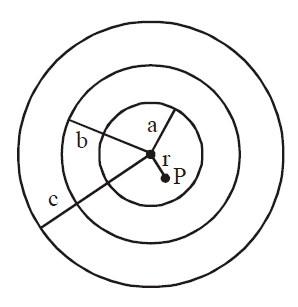# Q. A charge Q is distributed over three concentric spherical shells of radii a, b, c (a < b < c ) such that their surface charge densities are equal to one another. The total potential at a point at distance r from their common centre, where r < a, would be :

JEE Main JEE Main 2019

Solution:

## Potential at point P, $V = \dfrac{kQ_{a}}{a} + \dfrac{kQ_{b}}{b} + \dfrac{kQ_{c}}{c}$ $\because Q_{a} : Q_{b} : Q_{c} : : a^{2} :b^{2} :c^{2}$ $\left\{\text{since} \sigma_{a} = \sigma_{b} =\sigma_{c}\right\}$ $\therefore Q_{a} = \left[\dfrac{a^{2}}{a^{2}+b^{2}+c^{2}}\right]Q$ $Q_{b} = \left[\dfrac{b^{2}}{a^{2}+ b^{2} +c^{2}}\right]Q$ $Q_{c} = \left[\dfrac{c^{2}}{a^{2}+b^{2} +c^{2}}\right]Q$ $V = \dfrac{Q}{4 \pi\in_{0}} \left[\dfrac{\left(a+b+c\right)}{a^{2}+b^{2}+c^{2}}\right]$ $\therefore$ correct answer is (2)You must select option to get answer and solution

## 1. The increase in pressure required to decrease the 200 L volume of a liquid by 0.004% (in kPa) is (Bulk modulus of the liquid ==2100 MPa)

Maharashtra CET 2006 Mechanical Properties Of Solids

## 2. To have large selectivity in a series LCR circuit

COMEDK 2006 Alternating Current

## 3. The electric field and the potential of an electric dipole vary with distance r as

Manipal 1980 Electric Charges And Fields

## 4. To draw maximum current from a combination of cells, how should the cells be grouped?

AFMC 2006 Current Electricity

NEET 2019 Waves

AFMC 2010 Atoms

## 7. The period of revolution of planet A around the sun is 8 times that of B. The distance of A from the sun is how many times greater than that of B from the sun?

AIPMT 1997 Gravitation

## 8. The temperature of 100 g of water is to be raised from 24°C to 90°C by adding steam to it. The mass of the steam required for this purpose is

JIPMER 2015 Thermal Properties Of Matter

## 9. Two discs of same moment of inertia rotating about their regular axis passing through centre and perpendicular to the plane of disc with angular velocities $\omega_1$ and $\omega_2$ . They are brought into contact face to face coinciding the axis of rotation. The expression for loss of energy during this process is:-

NEET 2017 System Of Particles And Rotational Motion

## 10. The hot wire ammeter measures

Rajasthan PMT 2004 Alternating Current